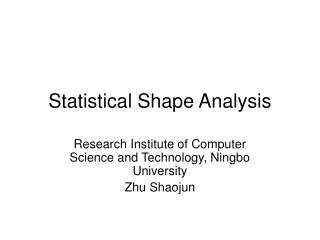# Statistical Shape Analysis - PowerPoint PPT PresentationDownload PresentationStatistical Shape Analysis

Statistical Shape AnalysisDownload Presentation## Statistical Shape Analysis

- - - - - - - - - - - - - - - - - - - - - - - - - - - E N D - - - - - - - - - - - - - - - - - - - - - - - - - - -
##### Presentation Transcript

1. Statistical Shape Analysis Research Institute of Computer Science and Technology, Ningbo University Zhu Shaojun

2. What is a shape? • Shape is all the geometrical information that remains when location, scale and rotational effects are filtered out from an object.

3. Landmark • A landmark is a point of correspondence on each object that matches between and within populations. • Anatomical landmarks • Mathematical landmarks • Pseudo-landmarks

4. Landmark • A mathematical representation of an n-point shape in k dimensions could be to concatenate each dimension into a kn-vector. The vector representation for planar shapes (i.e. k = 2) would then be:

5. Shape Alignment • To obtain a true shape representation – according to our definition – location, scale and rotational effects need to be filtered out.

6. The Procrustes Shape Distance • Compute the centroid of each shape. • Re-scale each shape to have equal size. • Align w.r.t. position the two shapes at their centroids. • Align w.r.t. orientation by rotation.

7. shape size metric • A shape size metric S(x) is any positive real valued function of the shape vector that fulfils the following property (like all other metrics):

8. shape size metric

9. rotation • Arrange the size and position aligned x1 and x2 as n*k matrices (in the planar case k = 2). • Calculate the SVD, UDV(T), of x1(T)x2 in order to maximize the correlation between the two sets of landmarks. • The rotation matrix needed to optimally superimpose x1 upon x2 is then VU(T):

10. Generalized Procrustes Analysis • Choose an initial estimate of the mean shape (e.g. the first shape in the set). • Align all the remaining shapes to the mean shape. • Re-calculate the estimate of the mean from the aligned shapes. • If the estimated mean has changed return to step 2.